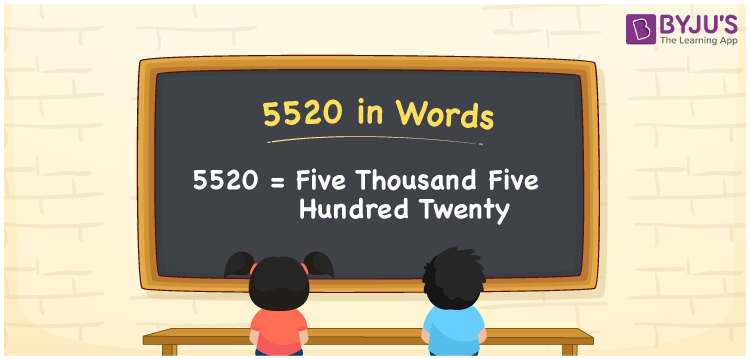# 5520 in Words

5520 in words is Five thousand five hundred twenty. In general, we use the word form of the numbers to express or read that particular number. Suppose the number 5 can be spelt as five, 2 can be spelt as two, and so on. Similarly, we can express 5520 as Five thousand five hundred twenty. However, writing the word form of numbers less than 100 is easy when compared to large numbers. To overcome this, we can make use of the place value chart. In this article, you will learn how to convert the number 5520 into words in detail.

 5520 in words Five thousand five hundred twenty Five thousand five hundred twenty in Numbers 5520

## 5520 in English Words

With the help of the English alphabet, we generally express numbers in words. So, we can spell 5520 in English as “Five thousand five hundred twenty”.## How to Write 5520 in Words?

In this section, you will understand how to write the number 5520 in words with the help of a value chart. The below table shows the place values for all the digits of 5520.

 Thousands Hundreds Tens Ones 5 5 2 0

Here, ones = 0, tens = 2, hundreds = 5, and thousands = 5.

Thus, we can write the above digits with respect to their place values as given below.

5 × Thousand + 5 × Hundred + 2 × Ten + 0 × One

= 5 × 1000 + 5 × 100 + 2 × 10 + 0 × 1

= 5000 + 500 + 20

= Five thousand + Five hundred + Twenty

= Five thousand five hundred twenty

Therefore, 5520 in words = Five thousand five hundred twenty.

Also, check: place value

5520 is a natural number that precedes 5521 and succeeds 5519.

5520 in words – Five thousand five hundred twenty

Is 5520 an odd number? – No

Is 5520 an even number? – Yes

Is 5520 a prime number? – No

Is 5520 a composite number? – Yes

Is 5520 a perfect square number? – No

Is 5520 a perfect cube number? – No

## Frequently Asked Questions on 5520 in Words

Q1

### Write 5520 in words.

The number 5520 can be written in words as Five thousand five hundred twenty.
Q2

### How to write an amount of Rs. 5520 in words on a cheque?

On a cheque, an amount of Rs. 5520 can be expressed in words as “Five thousand five hundred twenty rupees only.
Q3

### What number is Five thousand five hundred twenty in words?

As we know, 5520 is spelt as Five thousand five hundred twenty in words.